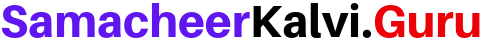# Samacheer Kalvi 7th Maths Solutions Term 1 Chapter 1 Number System Ex 1.4

Students can Download Maths Chapter 1 Number System Ex 1.4 Questions and Answers, Notes Pdf, Samacheer Kalvi 7th Maths Book Solutions Guide Pdf helps you to revise the complete Tamilnadu State Board New Syllabus and score more marks in your examinations.

## Tamilnadu Samacheer Kalvi 7th Maths Solutions Term 1 Chapter 1 Number System Ex 1.4

Question 1.
Fill in the blanks.
(i) (-40) ÷ ___ =40
(ii) 25 ÷ ____ = -5
(iii) ____ ÷ (-4) = 9
(iv) (-62) ÷ (-62) = ____
Solution:
(i) -1
(ii) -5
(iii) -36
(iv) 1

Question 2.
Say True or False:
(i) (-30) ÷ (-6) = -6
(ii) (-64) ÷ (-64) is 0
Solution:
(i) False
(ii) False

Question 3.
Find the values of the following.
(i) (-75) ÷ 5
(ii) (-100) ÷ (-20)
(iii) 45 ÷ (-9)
(iv) (-82) ÷ 82
Solution:
(i) $$\frac{-75}{5}$$ = -15
(ii) $$\frac{-100}{-20}$$ = 5
(iii) $$\frac{45}{-9}$$ = -5
(iv) $$\frac{-82}{82}$$ = -1

Question 4.
The product of two integers is -135. If one number is -15. Find the other integer.
Solution:
Given the product of two integers = -135
One of them = -15
∴ -15 × Another number = -135
Other number = $$\frac{-135}{-15}$$ = 9
∴ The other number = 9.Question 5.
In 8 hours duration, with uniform decrease in temperature, the temperature dropped 24°C. How many degrees did the temperature drop each hour?
Solution:
In 8 hours the drop in temperature = 24
In 1 hour the drop in temperature = $$\frac{24}{8}$$ = 3°
The temperature dropped 3°C every hour.

Question 6.
An elevator descends into a mine shaft at the rate of 5 m/min. If the descent starts from 15 m above the ground level, how long will it take to reach -250 m?
Solution:
The elevator’s position = 15 m above ground level = +15 m
It should reach = -250 m
The distance to be travelled = 15 – (-250) m = 15 + (+250) m 265 m
Time taken to descend 5 m = 1 min
∴ Time required to descend 265 m = $$\frac{24}{8}$$ = 53 min

Question 7.
A person lost 4800 calories in 30 days. If the calory loss is uniform, calculate the loss of calory per day.
Solution:
Loss of calory in 30 days = 4800
∴ Loss of calory in 1 day = $$\frac{4800}{30}$$ = 160 calories
∴ 160 calories lost per day.

Question 8.
Given 168 × 32 = 5376 then fined (-5376) ÷ (-32).
Solution:
Given 168 × 32 = 5376
∴ $$\frac{5376}{32}$$ = 168
Also, $$\frac{-5376}{-32}$$ = 168Question 9.
How many -4’s are there is (-20)?
Solution:
Number of -4’s in (-20) = $$\frac{-20}{-4}$$ = 5

Question 10.
(-400) divided into 10 equal parts gives ____
Solution:
$$\frac{-400}{10}$$ = -4

Objective Type Questions

Question 11.
Which of the following does not represent an integer?
(i) 0 ÷ (-7)
(ii) 20 ÷ (-4)
(iii) (-9) ÷ 3
(iv) 12 ÷ 5
Solution:
(iv) 12 ÷ 5

Question 12.
(-16) ÷ 4 is the same as
(i) -(-16 ÷ -4)
(ii) -(16) ÷ (-4)
(iii) 16 ÷ (-4)
(iv) -4 ÷ -16
Solution:
(iii) 16 ÷ (-4)Question 13.
(-200) ÷ 10 is
(i) 20
(ii) -20
(iii) -190
(iv) 210
Solution:
(ii) 20

Question 14.
The set of integers is not closed under